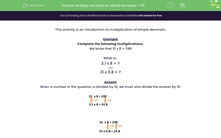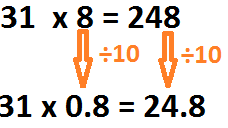# Multiply Decimals by Whole Numbers: 1-99

In this worksheet, students practise simple multiplication of decimals.Key stage:  KS 2

Curriculum topic:   Number: Multiplication and Division

Curriculum subtopic:   Multiply/Divide Using Decimals

Difficulty level:#### Worksheet Overview

This activity is an introduction to multiplication of simple decimals.

Example

Complete the following multiplications.

We know that 31 x 8 = 248

What is:

3.1 x 8 = ?

and

31 x 0.8 = ?

When a number in the question is divided by 10, we must also divide the answer by 10.